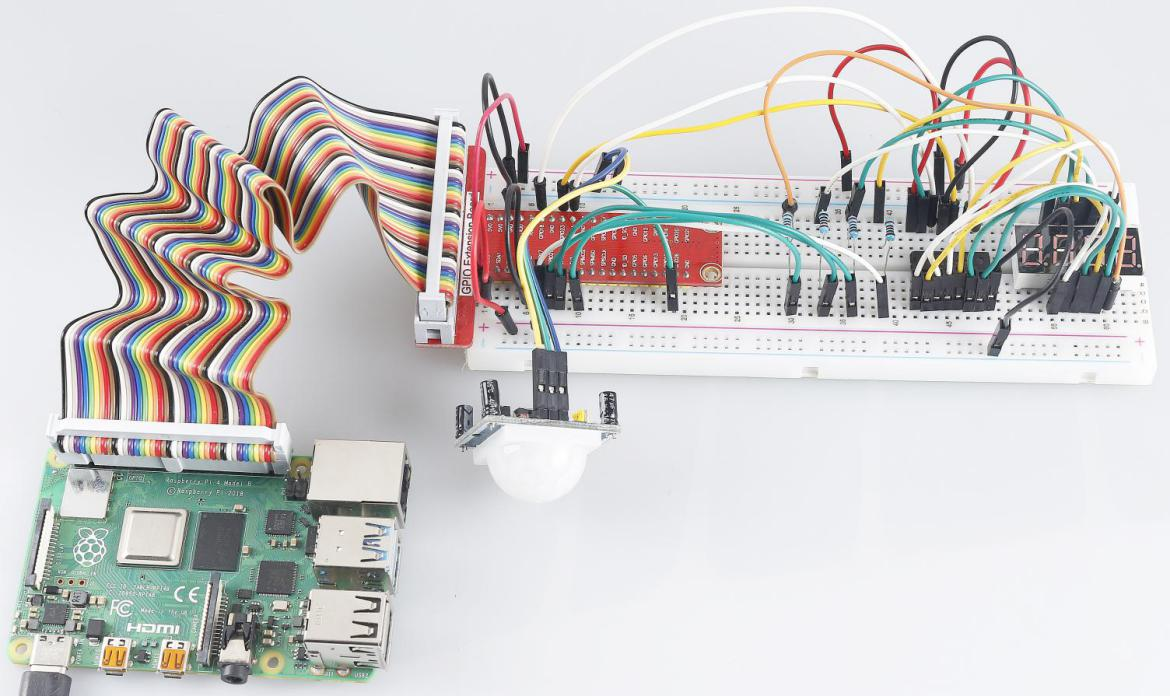# 3.1.1 Counting Device¶

## Introduction¶

Here we will make a number-displaying counter system, consisting of a PIR sensor and a 4-digit segment display. When the PIR detects that someone is passing by, the number on the 4-digit segment display will add 1. You can use this counter to count the number of people walking through the passageway.

## Components¶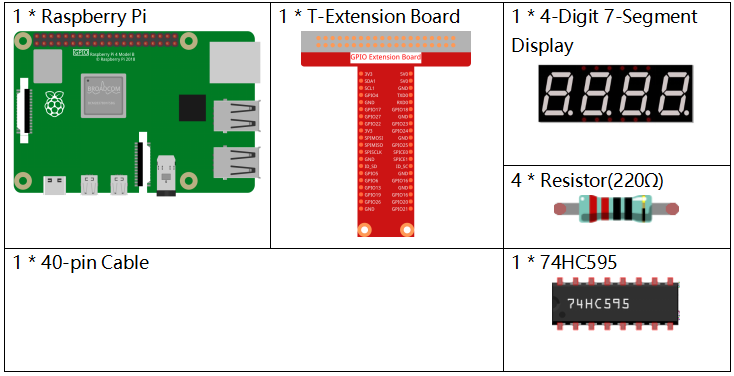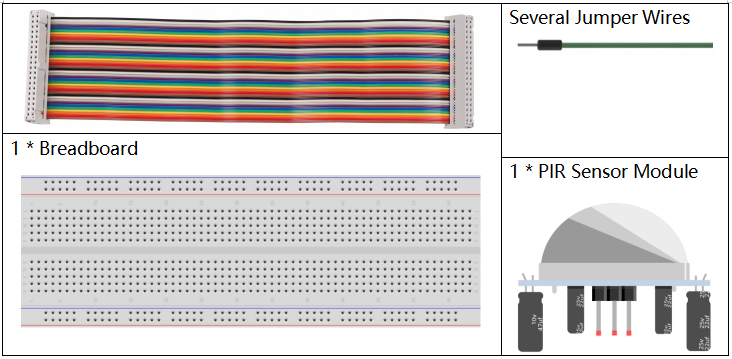## Schematic Diagram¶

 T-Board Name physical wiringPi BCM GPIO17 Pin 11 0 17 GPIO27 Pin 13 2 27 GPIO22 Pin 15 3 22 SPIMOSI Pin 19 12 10 GPIO18 Pin 12 1 18 GPIO23 Pin 16 4 23 GPIO24 Pin 18 5 24 GPIO26 Pin 37 25 26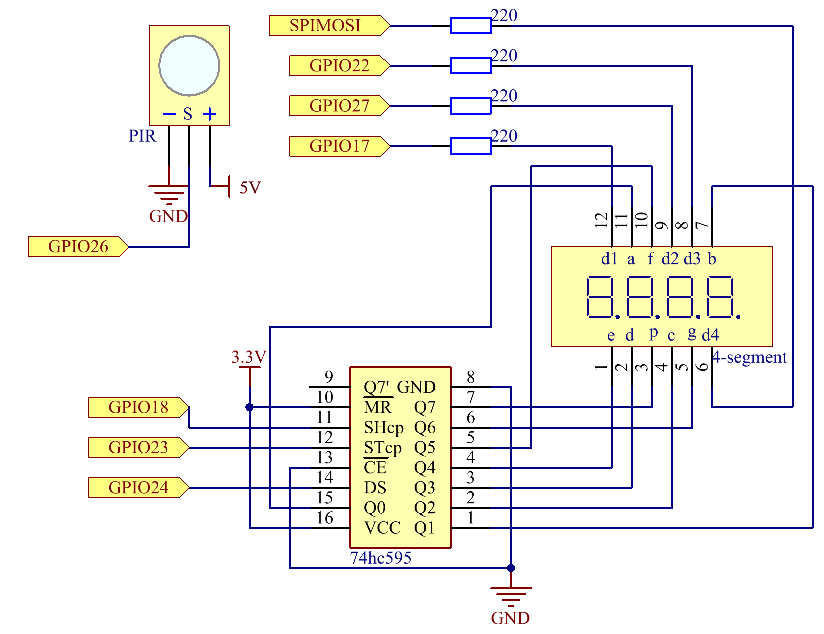## Experimental Procedures¶

Step 1: Build the circuit.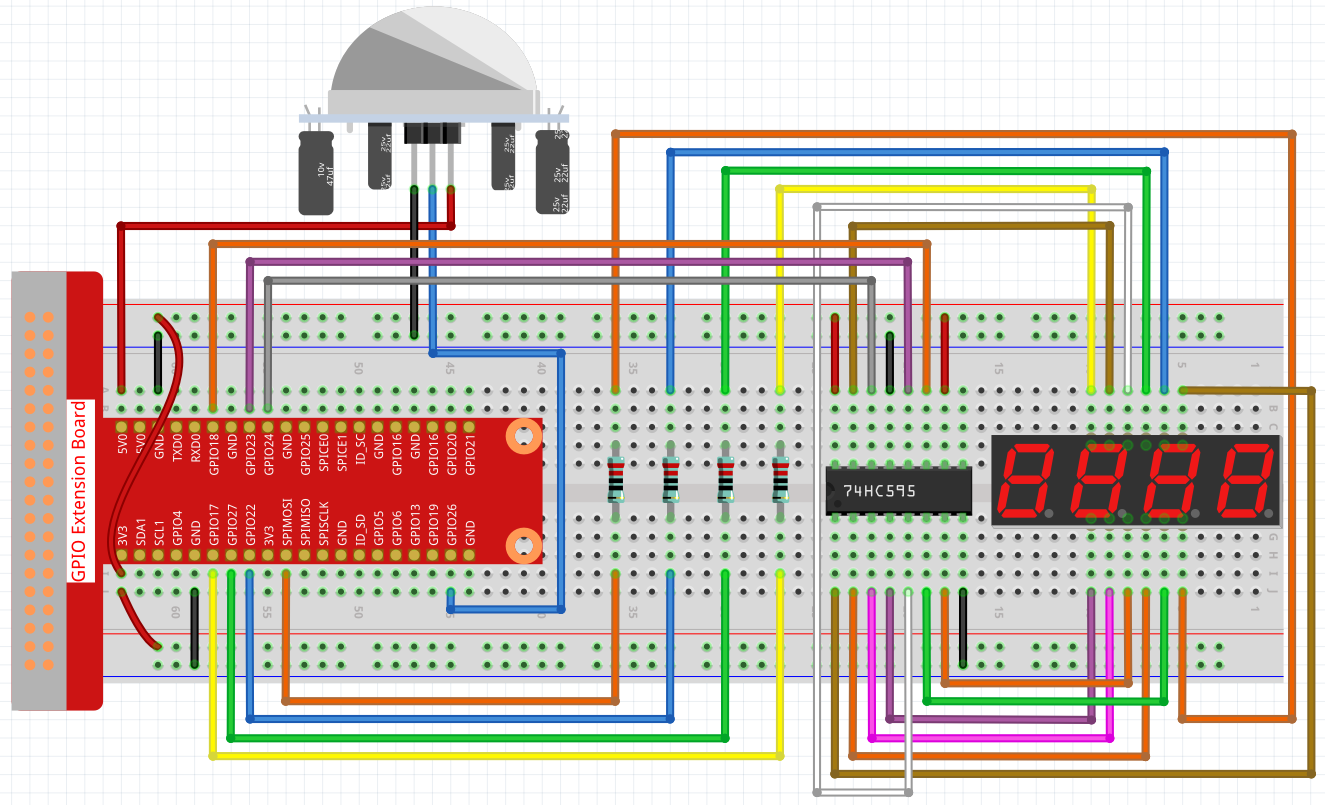### For C Language Users¶

Step 2: Go to the folder of the code.

```cd ~/davinci-kit-for-raspberry-pi/c/3.1.1/
```

Step 3: Compile the code.

```gcc 3.1.1_CountingDevice.c -lwiringPi
```

Step 4: Run the executable file.

```sudo ./a.out
```

After the code runs, when the PIR detects that someone is passing by, the number on the 4-digit segment display will add 1.

There are two potentiometers on the PIR module: one is to adjust sensitivity and the other is to adjust the detection distance. To make the PIR module work better, you You need to turn both of them counterclockwise to the end.

Note

If it does not work after running, or there is an error prompt: "wiringPi.h: No such file or directory", please refer to C code is not working?.

Code Explanation

```void display()
{
clearDisplay();
pickDigit(0);
hc595_shift(number[counter % 10]);

clearDisplay();
pickDigit(1);
hc595_shift(number[counter % 100 / 10]);

clearDisplay();
pickDigit(2);
hc595_shift(number[counter % 1000 / 100]);

clearDisplay();
pickDigit(3);
hc595_shift(number[counter % 10000 / 1000]);
}
```

First, start the fourth segment display, write the single-digit number. Then start the third segment display, and type in the tens digit; after that, start the second and the first segment display respectively, and write the hundreds and thousands digits respectively. Because the refreshing speed is very fast, we see a complete four-digit display.

```void loop(){
int currentState =0;
int lastState=0;
while(1){
display();
if((currentState==0)&&(lastState==1)){
counter +=1;
}
lastState=currentState;
}
}
```

This is the main function: display the number on the 4-digit segment display and read the PIR value. When the PIR detects that someone is passing by, the number on the 4-digit segment display will add 1.

### For Python Language Users¶

Step 2: Go to the folder of the code.

```cd ~/davinci-kit-for-raspberry-pi/python/
```

Step 3: Run the executable file.

```sudo python3 3.1.1_CountingDevice.py
```

After the code runs, when the PIR detects that someone is passing by, the number on the 4-digit segment display will add 1.

code

Note

You can Modify/Reset/Copy/Run/Stop the code below. But before that, you need to go to source code path like `davinci-kit-for-raspberry-pi/python`.

```import RPi.GPIO as GPIO
import time

sensorPin = 26

SDI = 24
RCLK = 23
SRCLK = 18

placePin = (10, 22, 27, 17)
number = (0xc0, 0xf9, 0xa4, 0xb0, 0x99, 0x92, 0x82, 0xf8, 0x80, 0x90)

counter = 0

def clearDisplay():
for i in range(8):
GPIO.output(SDI, 1)
GPIO.output(SRCLK, GPIO.HIGH)
GPIO.output(SRCLK, GPIO.LOW)
GPIO.output(RCLK, GPIO.HIGH)
GPIO.output(RCLK, GPIO.LOW)

def hc595_shift(data):
for i in range(8):
GPIO.output(SDI, 0x80 & (data << i))
GPIO.output(SRCLK, GPIO.HIGH)
GPIO.output(SRCLK, GPIO.LOW)
GPIO.output(RCLK, GPIO.HIGH)
GPIO.output(RCLK, GPIO.LOW)

def pickDigit(digit):
for i in placePin:
GPIO.output(i,GPIO.LOW)
GPIO.output(placePin[digit], GPIO.HIGH)

def display():
global counter
clearDisplay()
pickDigit(0)
hc595_shift(number[counter % 10])

clearDisplay()
pickDigit(1)
hc595_shift(number[counter % 100//10])

clearDisplay()
pickDigit(2)
hc595_shift(number[counter % 1000//100])

clearDisplay()
pickDigit(3)
hc595_shift(number[counter % 10000//1000])

def loop():
global counter
currentState = 0
lastState = 0
while True:
display()
currentState=GPIO.input(sensorPin)
if (currentState == 0) and (lastState == 1):
counter +=1
lastState=currentState

def setup():
GPIO.setmode(GPIO.BCM)
GPIO.setup(SDI, GPIO.OUT)
GPIO.setup(RCLK, GPIO.OUT)
GPIO.setup(SRCLK, GPIO.OUT)
for i in placePin:
GPIO.setup(i, GPIO.OUT)
GPIO.setup(sensorPin, GPIO.IN)

def destroy():   # When "Ctrl+C" is pressed, the function is executed.
GPIO.cleanup()

if __name__ == '__main__':  # Program starting from here
setup()
try:
loop()
except KeyboardInterrupt:
destroy()
```

Code Explanation

Based on 1.1.5 4-Digit 7-Segment Display, this lesson adds PIR module to change the automatic counting of lesson 1.1.5 into count detecting. When the PIR detects that someone is passing by, the number on the 4-digit segment display will add 1.

```def display():
global counter
clearDisplay()
pickDigit(0)
hc595_shift(number[counter % 10])

clearDisplay()
pickDigit(1)
hc595_shift(number[counter % 100//10])

clearDisplay()
pickDigit(2)
hc595_shift(number[counter % 1000//100])

clearDisplay()
pickDigit(3)
hc595_shift(number[counter % 10000//1000])
```

First, start the fourth segment display, write the single-digit number. Then start the third segment display, and type in the tens digit; after that, start the second and the first segment display respectively, and write the hundreds and thousands digits respectively. Because the refreshing speed is very fast, we see a complete four-digit display.

```def loop():
global counter
currentState = 0
lastState = 0
while True:
display()
currentState=GPIO.input(sensorPin)
if (currentState == 0) and (lastState == 1):
counter +=1
lastState=currentState
```

This is the main function: display the number on the 4-digit segment display and read the PIR value. When the PIR detects that someone is passing by, the number on the 4-digit segment display will add 1.

## Phenomenon Picture¶